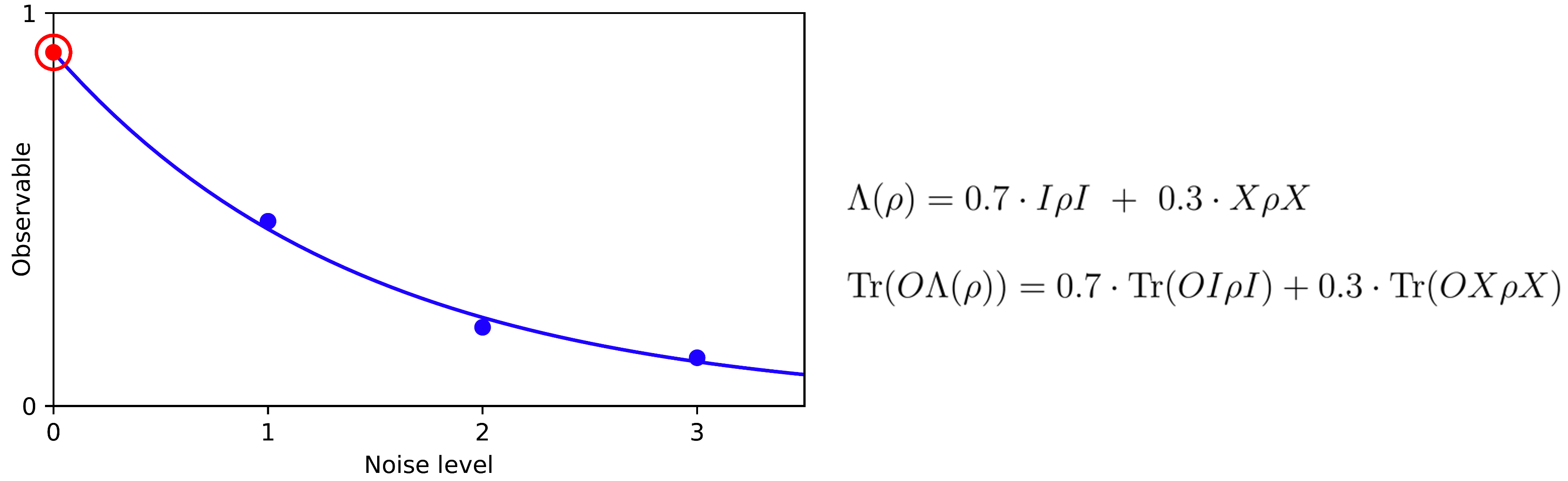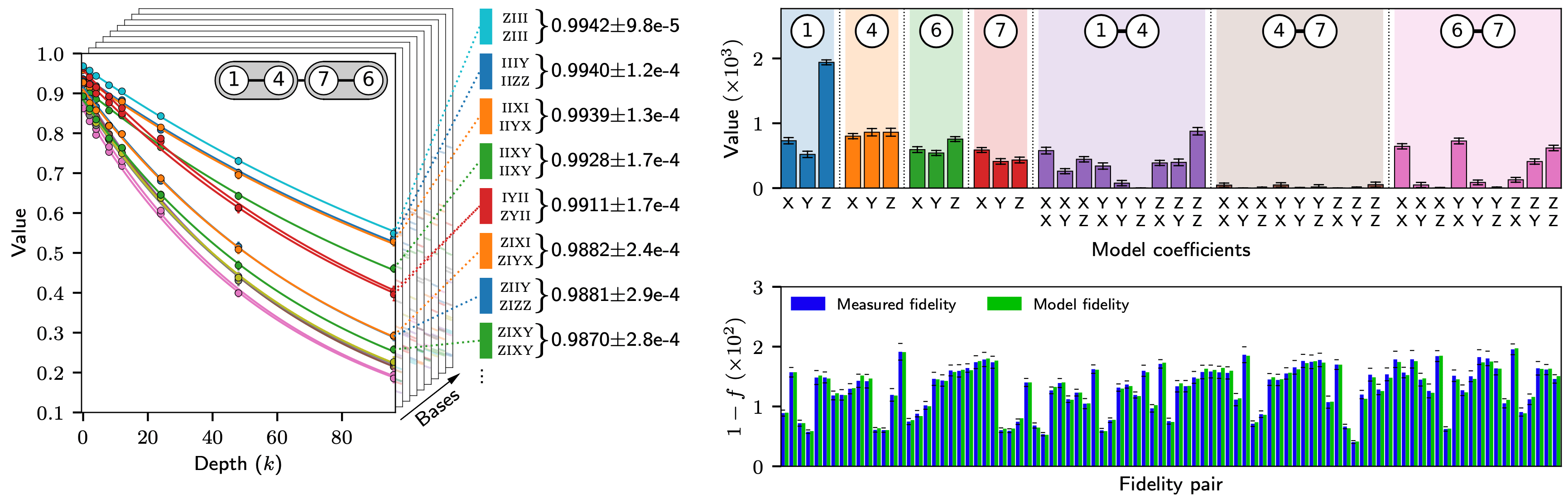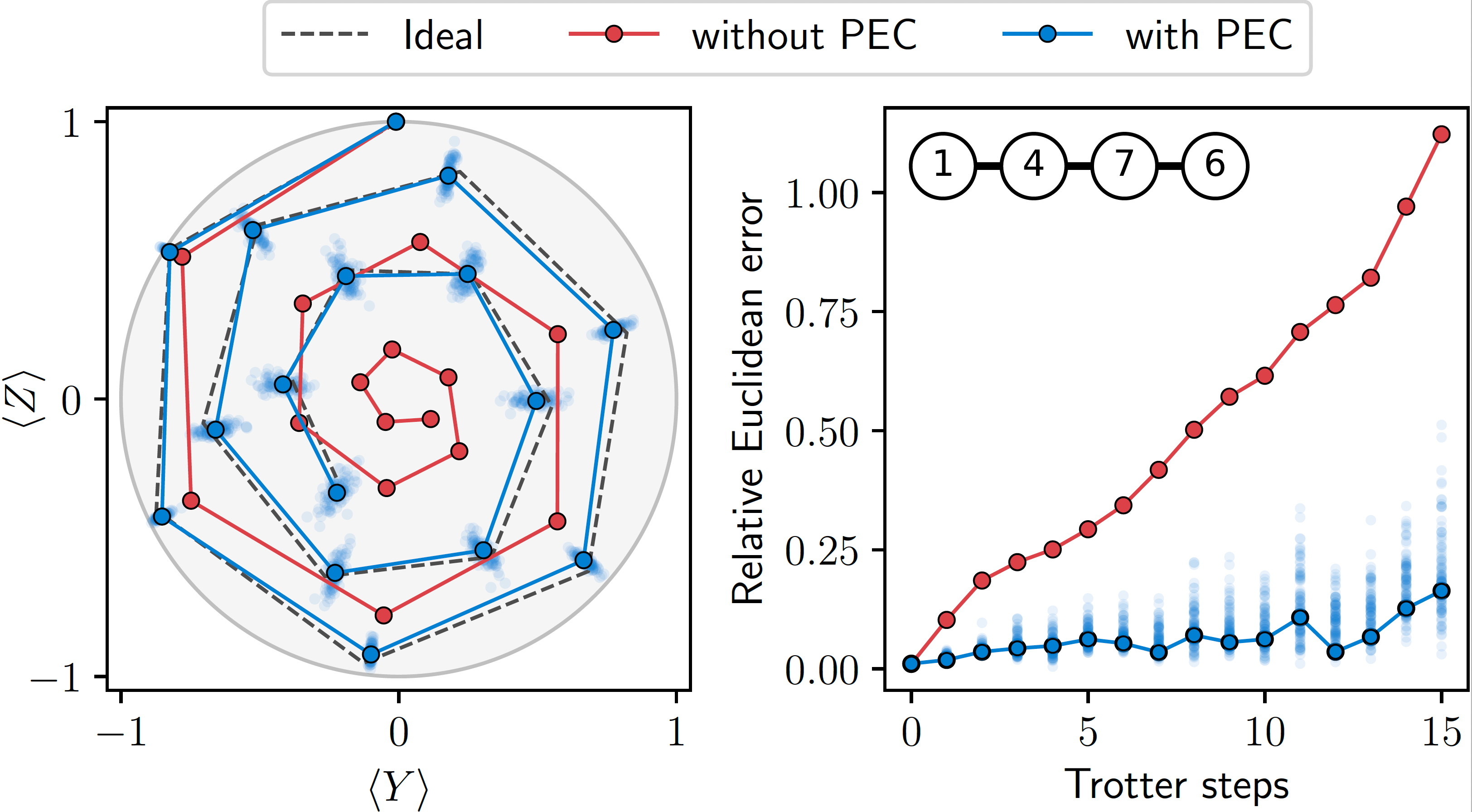# Probabilistic error cancellation with sparse Pauli-Lindblad noise models

Over the last several years we have witnessed incredible advances in quantum processor technology; the number of available qubits has risen from a just handful to well over a hundred and quantum gates can be applied more accurately than ever before. Nevertheless, remaining imperfections in the control and manufacturing of quantum processor units, as well as the very nature of open quantum systems themselves, mean that quantum circuit execution continues to be susceptible to noise. In the longer term, techniques from quantum error correction will be able to achieve a high level of fault tolerance, but this will require substantial further improvements in gate fidelity and qubit count. It should therefore come as no surprise that quantum error mitigation, aimed at getting the most out of contemporary quantum processors, is a highly active area of research.

The outcome of quantum circuits generally comes either in the form of individual shots, for instance in Shor's algorithm, or as expectation values of one or more observables, for instance when evaluating the ground-state energy of a molecule. The two main techniques for mitigating errors in the latter setting are zero-noise extrapolation (ZNE) and probabilistic error cancellation (PEC) [1,2]. Zero-noise extrapolation works by estimating the same observable at a set of different noise levels, followed by extrapolation to the zero-noise limit. This technique requires careful tuning of the noise, for instance by tuning control pulses to implement quantum gates with different durations, or digitally by repeatedly applying and undoing a gate before its actual application. ZNE applies quite generally to entire quantum circuits but is known to generally yield biased estimates. Probabilistic error cancellation, on the other hand, requires a more precise characterization of the gates, but does enable bias-free estimates. The core idea in PEC is to express an ideal operator as a linear combination of noisy operators. This linear combination can be expressed as a quasi-probability distribution according to which we can sample an ensemble of different quantum circuits. Leveraging the linearity of the observable expectation value, it then follows that the average of the individual observable estimates converges to the ideal value. One consequence of applying quasi-probabilistic sampling, however, is the need to scale up the estimated observable values by a scalar quantity that depends on the coefficients in the linear combination of operators. This scaling amplifies the estimator variance, which in turn translates into an increase in sample complexity.Figure 1 – Left: Illustration of the zero-noise error mitigation technique. Estimates of an observable are obtained at different noise levels (blue dots) and extrapolated to obtain an estimate at the zero-noise level (red marker). In this example, extrapolation is done by fitting an exponential function (blue line) through the data and evaluating it at the zero-noise level. Right: By leveraging linearity of the observable expectation value we can implement the given noise channel using instances of two circuit types; one with identity gates (sampled with probability 0.7) and one with X gates (sampled with probability 0.3).

In our work , we use quasi-probabilistic sampling to implement the inverse of the noise channel affecting a selected set of concurrent gates. Clearly, we can only determine the inverse noise map if we have an accurate representation of the noise channel itself. However, general noise channels are prohibitively expensive to learn beyond a few qubits and we therefore first shape the noise by applying Pauli twirling. Doing so simplifies the noise to a Pauli channel, which is more amenable to learning, but generally still requires an exponential number of parameters in the number of qubits. We therefore propose a sparse Pauli-Lindblad noise model that assumes that noise can be represented as a combination of independent low-weight errors. The model is expressed in the form of a Lindblad master equation whose jump operators are chosen to be low-weight Pauli operators supported on neighboring qubits (although the generators themselves are low-weight, they can combine to form high-weight noise terms). The resulting model can be represented using only a small number of parameters that essentially grows linearly in the number of qubits. We additionally develop an effectively learning algorithm that allows us to learn large-scale noise models. Finally, the proposed noise model has the extremely favorable property that it provides an implicit representation of the inverse noise channel. This allows us to efficiently sample operators from the desired distribution without explicitly generating an exponentially large number of sample probabilities.Figure 2 - The noise model is learned based on fidelity estimates. To obtain these, circuits with repetitions of the twirled layer of noisy gates are first measured in appropriate bases to obtain a collection of observable values at different depths. The fidelity of interest is then given by the slope of the exponential curve fitted to the data points associated with a given observable. Top right: using the measured fidelities we solve a nonnegative least-squares problem to obtain the model parameters. Bottom right: comparison between the measured fidelities and those given by the fitted noise model.

Learning noise in the context of gates presents some unique challenges, which we explain and address in the paper. We focus on layers of non-overlapping two-qubit Clifford gates such as controlled-not (CNOT) gates; a common component in many circuits. As an application we consider time evolution of a one-dimensional transverse-field Ising model. The time evolution of these models can be approximated using Trotterization. In our setting, this results in circuits in which two unique layers of CNOT gates are repeatedly applied. In order to apply PEC, it therefore suffices to learn the noise for both layers and then construct a large set of circuit instances in which the inverse noise channels are replaced by Pauli operators sampled according to the distribution associated with those channels. Running and measuring these circuits on a quantum processor results in shot counts that are then used to estimate various observable values. We are interested in the global magnetization (<X>, <Y>, <Z>) of the system and therefore measure in the three Pauli bases. In Figure 3, we show magnetization obtained with and without error mitigation as a function of the number of Trotter steps, along with the ideal trajectory. For clarity we display the projection of the magnetization onto the Y-Z Bloch plane; the X component does not deviate much from the plane. The error-mitigated result clearly follows the ideal spiral much more closely than the unmitigated result, which shrinks to the origin at a much faster pace.Figure 3 - Left: Global magnetization terms and for a four-qubit Ising model simulated using up to 15 Trotter steps, obtained by running circuits with and without PEC, along with the exact values (points are connected by lines for clarity). Right: The relative Euclidean distance to the exact solution for both approaches, as a function of the number of Trotter steps. The light-colored data points represent samples obtained by bootstrapping the data.

Although our sparse noise model is proposed in the context of PEC, it has potentially many other applications. For example, the ability to learn a noise model for a full quantum processor in a tractable manner is extremely useful when analyzing the performance of the different qubits and identify possible problems.  Oh, and remember the zero-noise extrapolation algorithm mentioned earlier? Here, we can leverage the noise model to accurately control the noise level of gates through partial mitigation of the errors, or even by amplifying the noise. In fact, this combination yields a powerful new technique, as reported in the recent Nature paper .

 K. Temme, S. Bravyi, and J. M. Gambetta, Error mitigation for short-depth quantum circuits, Phys. Rev. Lett. 119, 180509 (2017).

 Y. Li and S. C. Benjamin, Efficient variational quantum simulator incorporating active error minimization, Phys. Rev. X 7, 021050 (2017).

 E. van den Berg,  Z.K. Minev, A. Kandala, and K. Temme, Probabilistic error cancellation with sparse Pauli–Lindblad models on noisy quantum processors, Nat. Phys. (2023).

 Y. Kim, A., Eddins, S. Anand et al., Evidence for the utility of quantum computing before fault tolerance. Nature 618, 500–505 (2023).